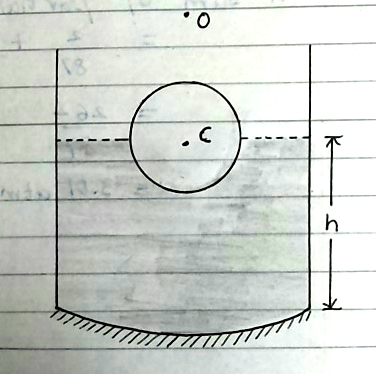# Optics... #1A transparent solid sphere of radius 2 cm, and density $\rho$ floats in a transparent liquid of density $2\rho$ kept in a beaker. The bottom of the beaker is spherical in shape with radius of curvature 8 cm and is silvered to make it a concave mirror as shown in the above figure. When an object is placed at a distance of 10 cm directly above the centre of the sphere C, its final image coincides with it. Find h (as in figure), the height of the liquid surface in the in the beaker from the apex of the bottom. Consider the paraxial rays only.

A paraxial ray is a ray that makes a small angle to the optical axis of the system, and lies close to the axis throughout the system.

Refractive index of sphere is $\frac{3}{2}$.

Refractive index of liquid is $\frac{4}{3}$.

Round that answer to nearest integer.

h is measured in cm